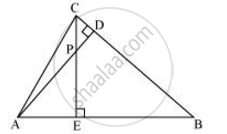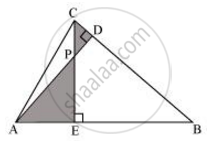# In the following figure, altitudes AD and CE of ΔABC intersect each other at the point P. Show that - Mathematics

In the following figure, altitudes AD and CE of ΔABC intersect each other at the point P. Show that:ΔAEP ∼ ΔCDP

#### SolutionIn ΔAEP and ΔCDP,

∠AEP = ∠CDP (Each 90°)

∠APE = ∠CPD (Vertically opposite angles)

Hence, by using AA similarity criterion,

ΔAEP ∼ ΔCDP

Concept: Criteria for Similarity of Triangles
Is there an error in this question or solution?

#### APPEARS IN

NCERT Class 10 Maths
Chapter 6 Triangles
Exercise 6.3 | Q 7.1 | Page 140